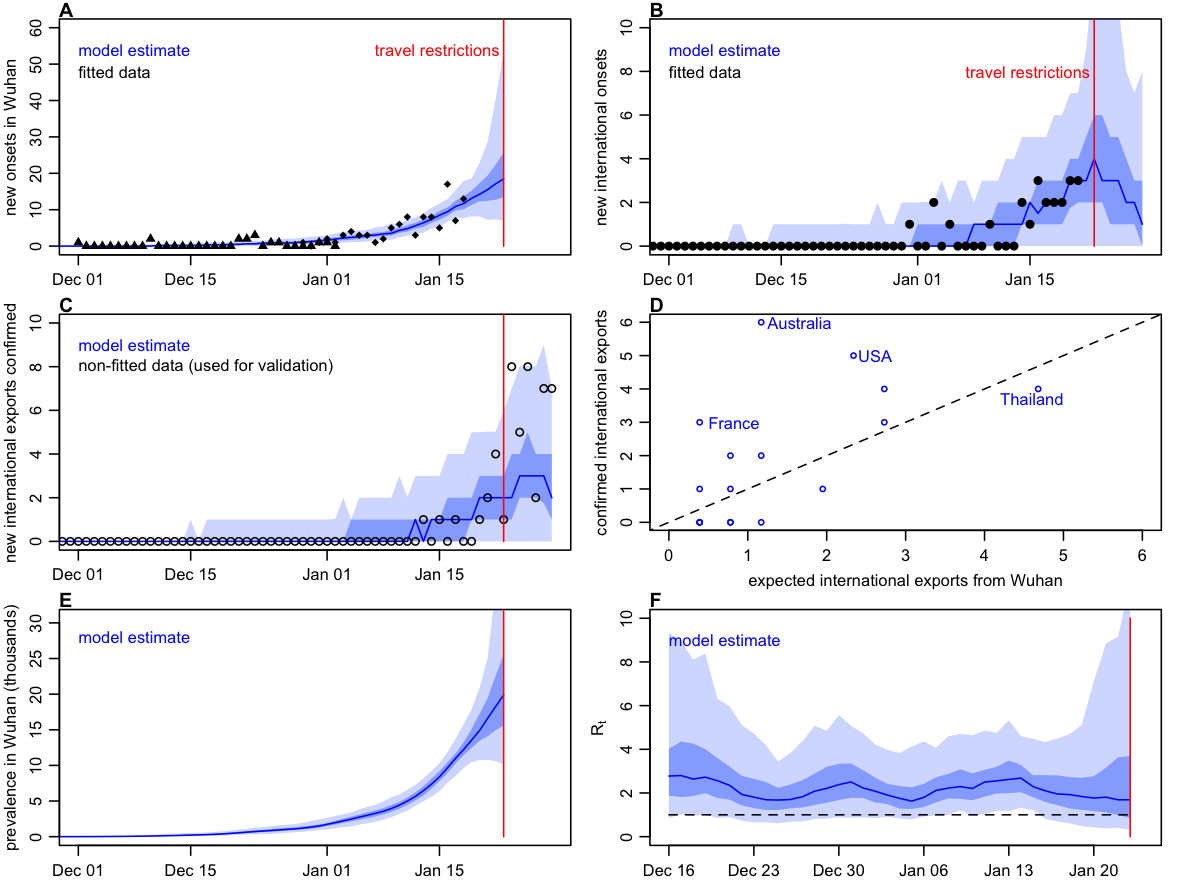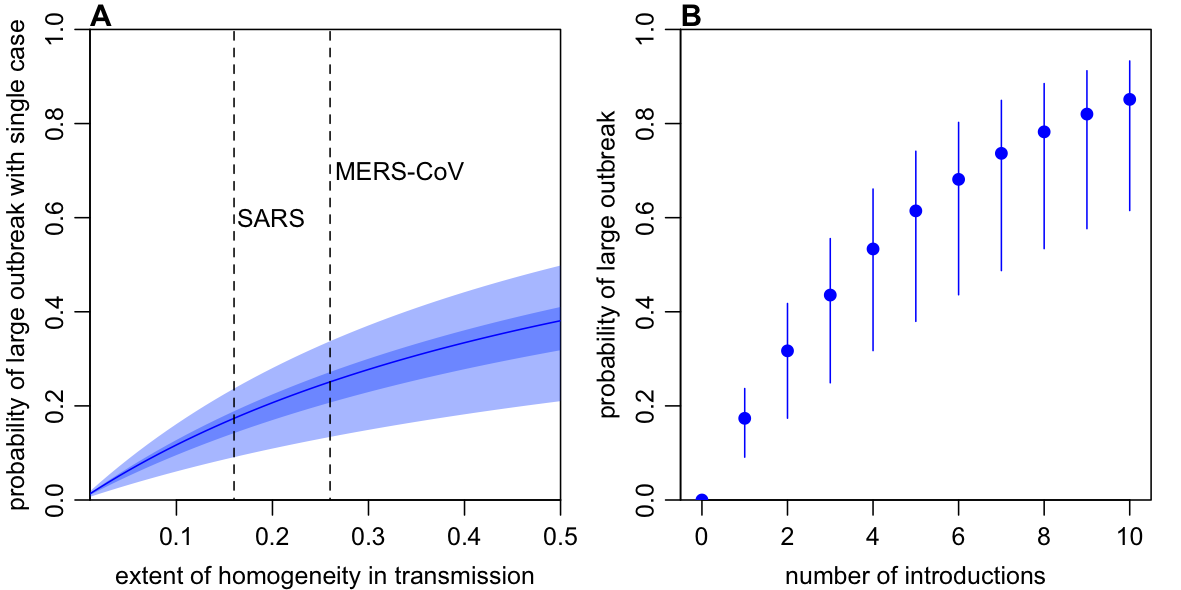Contributors: Adam Kucharski, Tim Russell, Charlie Diamond, CMMID nCoV working group, Sebastian Funk, Rosalind Eggo. Note: this is preliminary analysis and has not yet been peer-reviewed.

## Aim

To understand how human-to-human transmission varied in Wuhan during the early stages of the 2019-2020 nCoV outbreak, and implications for cases and outbreaks in new locations.

## Methods summary

• To estimate the early dynamics of transmission in Wuhan, we fitted a mathematical model to multiple available datasets on international exported cases from Wuhan and cases in Wuhan. Fitting to multiple data sources rather than a single dataset (or data point) is particularly useful for estimates in real-time, because some datasets may be unreliable.

• Transmission was a random process in the model, and could vary over time – this means the model can uncover fluctuations in transmission during the early stages of the outbreak. Our group previously used a similar analysis to understand the dynamics of Ebola in Liberia.

• We assumed that the chance of cases being exported from Wuhan to other countries depended on the number of cases in Wuhan, the number of outbound travellers (accounting for travel restrictions after 23rd January), and the relative connectivity of different countries. We considered the 20 countries outside China most at risk of exported cases in the analysis. The model accounts for delays in symptom onset and reporting (see methods below).

## Key results

We estimated that the median effective reproduction number, R, had likely been fluctuating between 1.6-2.9 prior to travel restrictions being introduced on 23rd Jan (Figure 1F). (The effective reproduction number is the average number of secondary cases generated by a typical infectious individual at a given point in time, assuming a fully susceptible population).

More confirmed cases with a link to Wuhan have been reported in USA, Australia and France than our model predicted (Figure 1D). This may be the result of increased surveillance and detection as awareness of 2019-nCoV grew in late January, which would suggest earlier exported cases may have missed; it may also be the result of increased travel out of Wuhan immediately prior to travel restrictions being introduced on 23rd January.

Based on the median reproduction number observed during January before travel restrictions were introduced, we estimated that a single introduction of 2019-nCoV with SARS-like or MERS-like individual-level variation in transmission would have a 20–28% probability of causing a large outbreak (Figure 2A). Assuming SARS-like variation and Wuhan-like transmission, we estimated that once more than three infections have been introduced into a new location, there is an over 50% chance that an outbreak will occur (Figure 2B).Figure 1: Dynamics of transmission in Wuhan, fitted up to 28 January 2020. Red line marks travel restrictions starting on 23 January 2020. A) Onset dates of confirmed cases in Wuhan (triangles) and China (diamonds). Blue lines and shaded regions: median, 50% and 95% credible intervals of model estimate. B) Reported cases by date of onset (black) and estimated internationally exported cases from Wuhan by date of onset (blue line). C) Cumulative confirmed cases by date in Wuhan (circles) and estimated cumulative cases (blue line). D) International exportation events by date of confirmation of case, and expected number of exports in the fitted model. E) Estimated prevalence of symptomatic cases over time in Wuhan. F) Estimated daily reproduction number (R) over time. Datasets that were fitted to shown as solid points; non-fitted data shown as circles.Figure 2: Risk that introduced infections will establish in a new population. A) Probability a single case will lead to a large outbreak for different assumptions about the extent of homogeneity in individual-level transmission (i.e. the dispersion parameter k in a negative binomial offspring process). Results are shown for the median reproduction number estimated for nCoV-2019 in Wuhan between 1st January and 23rd January 2020 and. B) Probability a given number of introductions will result in a large outbreak, assuming SARS-like superspreading events can occur.

## Discussion, caveats and ongoing work

• We used biological parameters from current papers, but these may change as we get better data. We also made assumptions about the proportion of people who travel following the methods in the J-IDEA reports. However, jointly fitting to multiple datasets will reduce the influence that one single dataset has on results.

• The cumulative case totals in Figure 1E should in theory only increase over time; the drop seen in early case is likely the result of reclassification of cases.

• We use connectivity estimates from MOBS lab for exported cases in our main analysis, but obtained similar results when using WorldPop data.

• The model is flexible, and this page will be updated as we incorporate new information and data.

## Detailed methods

#### Model

We used a stochastic SEIR model implemented using the Euler-Maruyama algorithm with a 6hr timestep, with transmission rate following geometric brownian motion (i.e. dlog(beta) = s dBt, where s is the volatility of transmission over time). We assume no travel out of Wuhan occurs after 23rd Jan, when restrictions were put in place. Cases that travel were distributed among other countries based on risk inferred from connectivity to those countries.